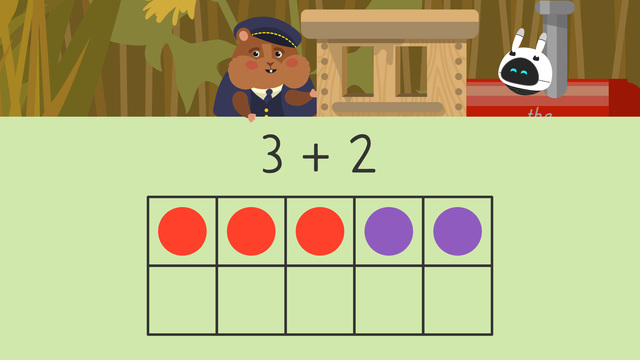# Using Ten Frames to Add

Content Using Ten Frames to AddRating

Ø 4.4 / 5 ratings
The authorsTeam Digital
CCSS.MATH.CONTENT.1.OA.C.6

## Basics on the topicUsing Ten Frames to Add

Ten frames are charts with two equal rows of five. They have ten spaces in total. When we place counters in those spaces, we can see different numbers within ten. Ten frames are useful to illustrate additions to twenty. For example, the number six has six counters in a ten frame. Each box has one marker, or counter, and there are six in all.If we fill up the entire ten frame, it means we have a set of ten! When we see a full ten frame, we know the amount is ten right away without having to count!We can use a ten frame horizontally or vertically. It works the same both ways because the amount of spaces does not change.## Addition with Ten Frames – Example

Why do we use ten frames in addition? Modeling addition using a ten frame helps children visualize the numbers you are adding together. Since each number is represented on the ten frame, it allows children to count out their answers.

Let’s use the example three plus two. Start by showing the first number, three, in counters on the ten frame.Then, show the second number, two, on the ten frame using counters again.Now, count ALL the filled spaces on the ten frame.There are five counters in all, so three train cars plus two train cars equals five train cars.## Ten Frames Addition – Summary

Ten frames can help you when you are doing additions up to 20. In order to visualize addition using ten frames, you need to do the following:

Step # What to do
1 Look at the first number in the problem and
put that many counters on the ten frame.
2 Then, look at the second number and put
that many more counters on.
3 Finally, count all the filled spaces on
the ten frame and write the answer.
• If a ten frame is full, you know you have ten without needing to count.
• If you fill up one ten frame, and still have more counters left, use another ten frame.

Have you practiced yet? After watching the video, you can also find interactive exercises, worksheets and more activities on ten frame addition.

## Using Ten Frames to Add exercise

Would you like to apply the knowledge you’ve learned? You can review and practice it with the tasks for the video Using Ten Frames to Add.
• ### Can you complete the addition number sentence?

Hints

How many red cars are there? This is the first number.

How many yellow cars are there? This is the second number.

How many spots are there altogether in the tens frame? This is the answer.

Solution

3 + 4 = 7

There were 3 red cars so we put 3 in the first box. There were 4 yellow cars so we put 4 in the second box. In total, there are 7 spots in the tens frame therefore there are 7 cars altogether.

As this is an addition problem, it does not matter which order the addends, or numbers we are adding, go.

We could have also put 4 + 3 = 7.

• ### How many altogether?

Hints

Look at the shape the dots are making to think about which number they are representing. Remember, if the tens frame is full we know that it equals 10.

For example, here we have 2 red dots and 2 yellow dots which is 4 altogether.

How many dots are there in each tens frame altogether?

Solution
• 2 + 4 = 6
• 4 + 5 = 9
• 1 + 7 = 8
• 7 + 3 = 10
• ### Which tens frame can help us solve the problem?

Hints

Which two numbers are we adding together? Which tens frame shows these two numbers?

How many red cars are there? This is the number of red dots there should be in the tens frame.

How many yellow cars are there? This is the number of yellow dots there should be in the tens frame.

Solution

This is the correct tens frame as there are:

• 2 red dots to represent the 2 red cars
• 7 yellow dots to represent the 7 yellow cars
We can see that it is adding 2 + 7 or 7 + 2.

• ### Use the tens frames to find the answers.

Hints

How many of each color dots are in each picture? These are the numbers you are looking for in the number sentence.

How many dots are there altogether in the tens frames? This is the answer to the number sentence.

For example, here we have:

• 10 red dots
• 7 purple dots
• 17 dots altogether
Therefore the number sentence would be 10 + 7 = 17.

Solution

Here we can see that:

• 6 + 6 = 12
• 10 + 6 = 16
• 6 + 8 = 14
• 10 + 10 = 20

• ### How many cars on the train?

Hints

We are adding 3 and 5 together so the answer will be bigger than either of these numbers.

Look at the tens frame, how many dots altogether?

Solution

Mr. Squeaks has 8 cars altogether on this train.

• 5 + 3 = 8
• 3 + 5 = 8
• ### What is the total in each of these tens frames?

Hints

Look at the amount of spaces the dots take up, which number is this equal to?

How many dots are in each set of tens frames altogether?

Solution

Here we can see the combinations that total each of the numbers.